# Surveying I Lecture 1 Sz Rzsa Surveying I

• Slides: 39Surveying I. Lecture 1. Sz. Rózsa: Surveying I. – Lecture 1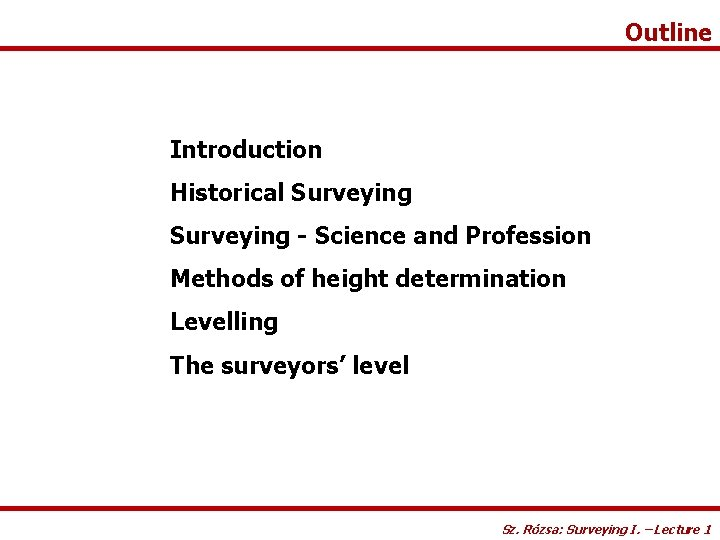Outline Introduction Historical Surveying - Science and Profession Methods of height determination Levelling The surveyors’ level Sz. Rózsa: Surveying I. – Lecture 1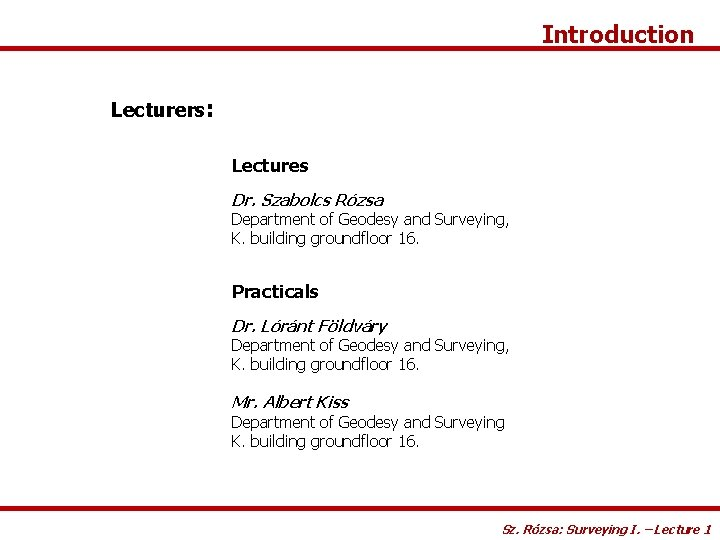Introduction Lecturers: Lectures Dr. Szabolcs Rózsa Department of Geodesy and Surveying, K. building groundfloor 16. Practicals Dr. Lóránt Földváry Department of Geodesy and Surveying, K. building groundfloor 16. Mr. Albert Kiss Department of Geodesy and Surveying K. building groundfloor 16. Sz. Rózsa: Surveying I. – Lecture 1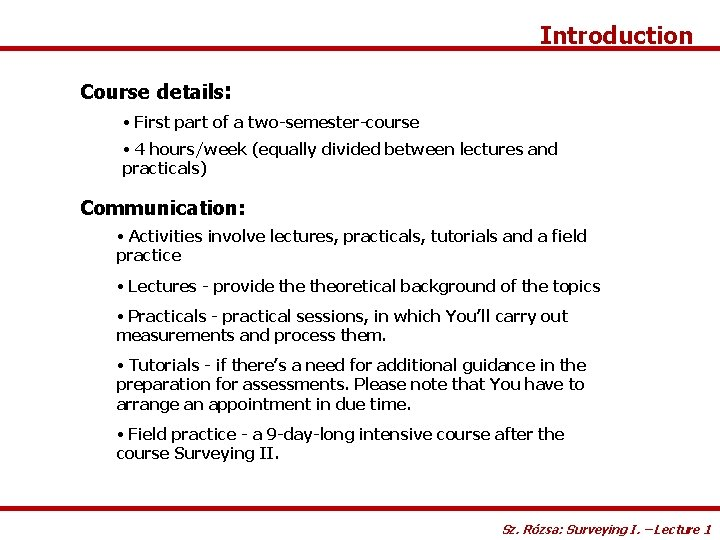Introduction Course details: • First part of a two-semester-course • 4 hours/week (equally divided between lectures and practicals) Communication: • Activities involve lectures, practicals, tutorials and a field practice • Lectures - provide theoretical background of the topics • Practicals - practical sessions, in which You’ll carry out measurements and process them. • Tutorials - if there’s a need for additional guidance in the preparation for assessments. Please note that You have to arrange an appointment in due time. • Field practice - a 9 -day-long intensive course after the course Surveying II. Sz. Rózsa: Surveying I. – Lecture 1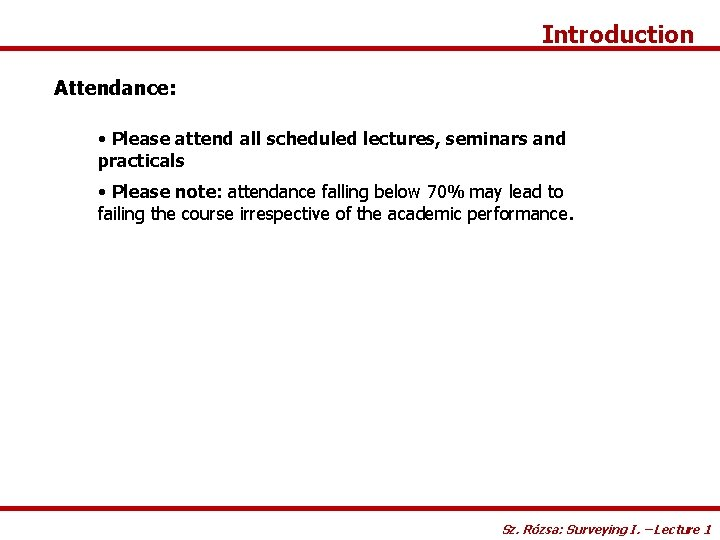Introduction Attendance: • Please attend all scheduled lectures, seminars and practicals • Please note: attendance falling below 70% may lead to failing the course irrespective of the academic performance. Sz. Rózsa: Surveying I. – Lecture 1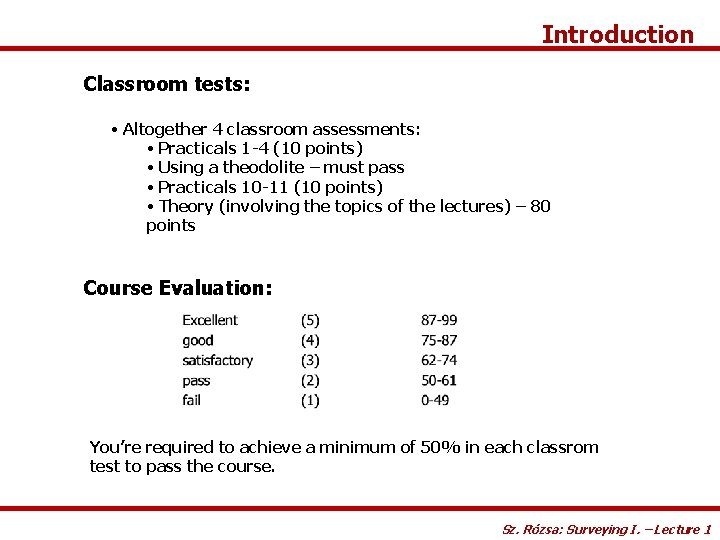Introduction Classroom tests: • Altogether 4 classroom assessments: • Practicals 1 -4 (10 points) • Using a theodolite – must pass • Practicals 10 -11 (10 points) • Theory (involving the topics of the lectures) – 80 points Course Evaluation: You’re required to achieve a minimum of 50% in each classrom test to pass the course. Sz. Rózsa: Surveying I. – Lecture 1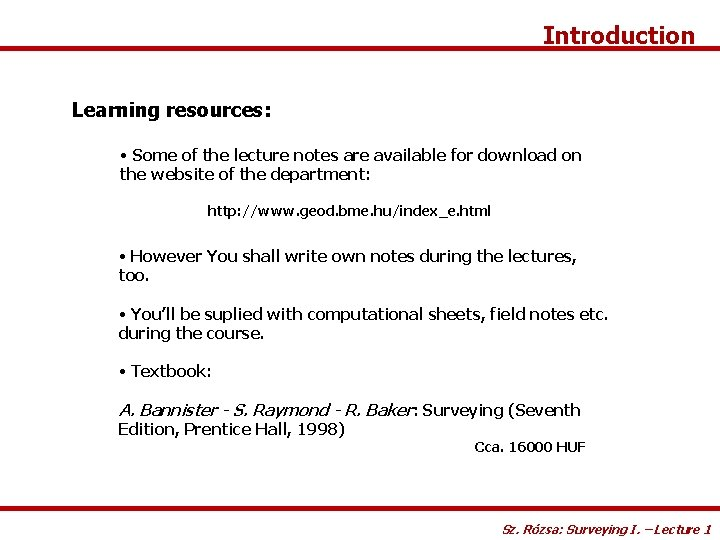Introduction Learning resources: • Some of the lecture notes are available for download on the website of the department: http: //www. geod. bme. hu/index_e. html • However You shall write own notes during the lectures, too. • You’ll be suplied with computational sheets, field notes etc. during the course. • Textbook: A. Bannister - S. Raymond - R. Baker: Surveying (Seventh Edition, Prentice Hall, 1998) Cca. 16000 HUF Sz. Rózsa: Surveying I. – Lecture 1Website Lecture notes can be downloaded from: http: //www. geod. bme. hu/index_e. html Sz. Rózsa: Surveying I. – Lecture 1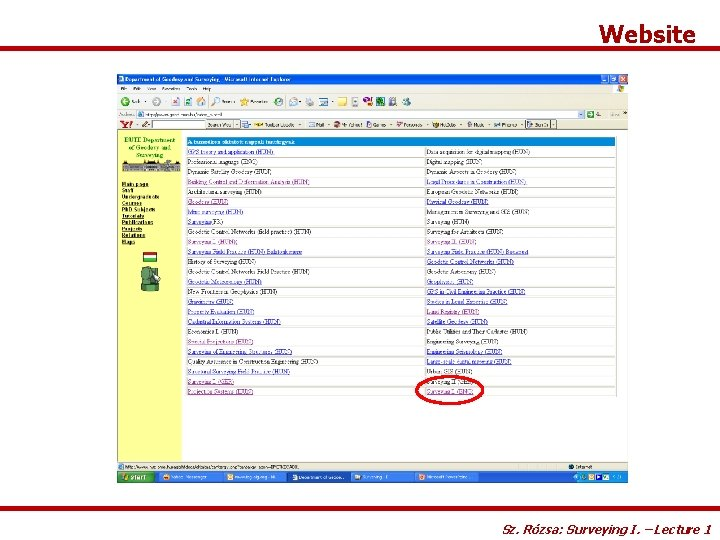Website Sz. Rózsa: Surveying I. – Lecture 1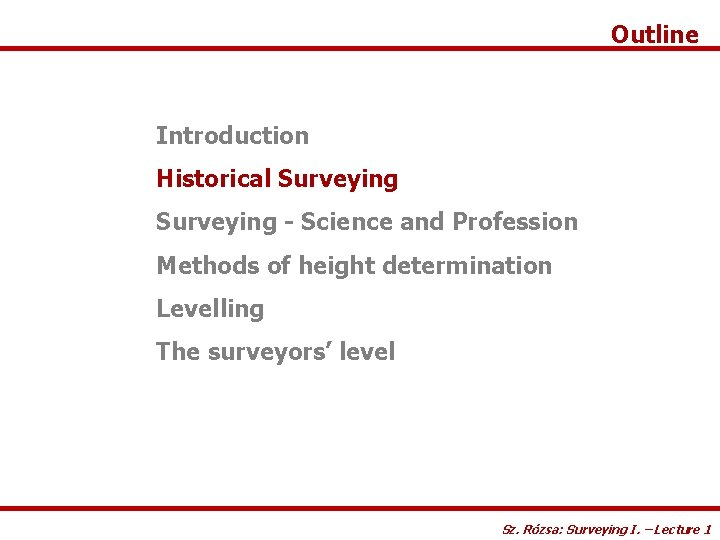Outline Introduction Historical Surveying - Science and Profession Methods of height determination Levelling The surveyors’ level Sz. Rózsa: Surveying I. – Lecture 1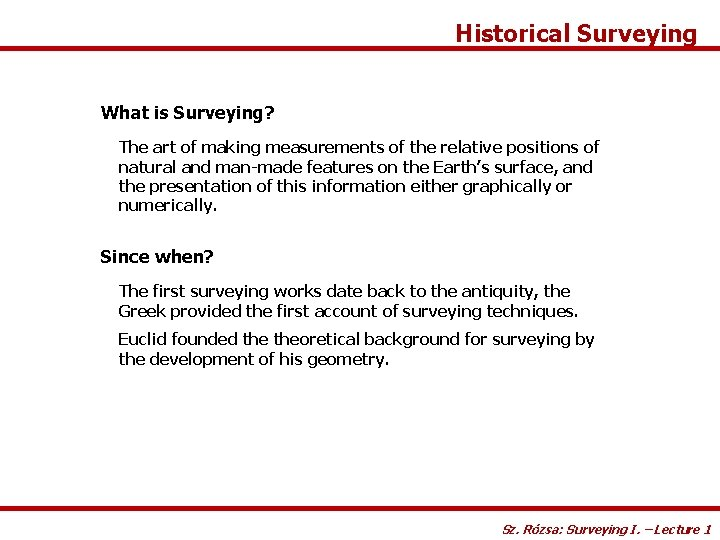Historical Surveying What is Surveying? The art of making measurements of the relative positions of natural and man-made features on the Earth’s surface, and the presentation of this information either graphically or numerically. Since when? The first surveying works date back to the antiquity, the Greek provided the first account of surveying techniques. Euclid founded theoretical background for surveying by the development of his geometry. Sz. Rózsa: Surveying I. – Lecture 1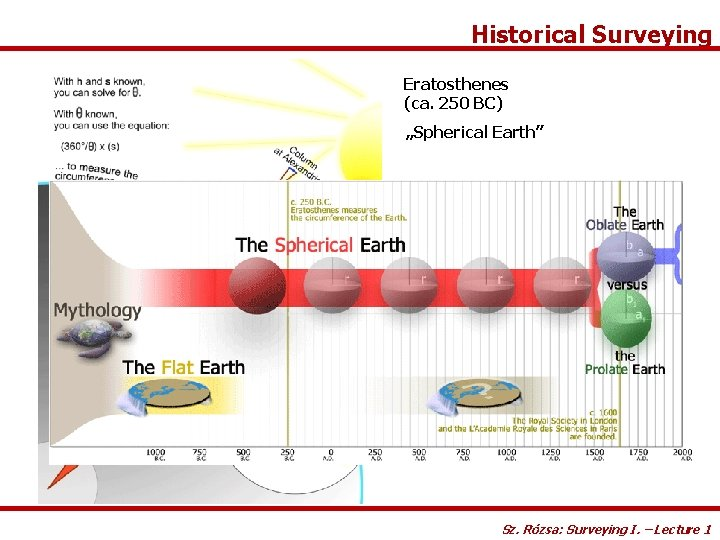Historical Surveying Eratosthenes (ca. 250 BC) „Spherical Earth” Sz. Rózsa: Surveying I. – Lecture 1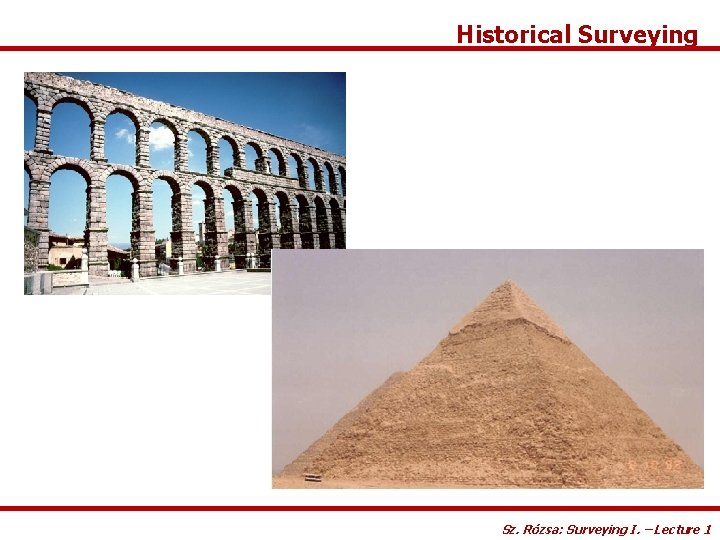Historical Surveying Sz. Rózsa: Surveying I. – Lecture 1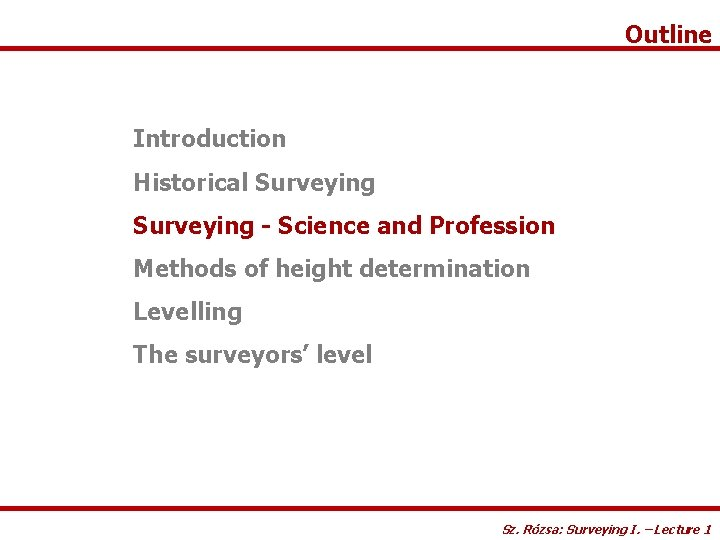Outline Introduction Historical Surveying - Science and Profession Methods of height determination Levelling The surveyors’ level Sz. Rózsa: Surveying I. – Lecture 1Surveying - Science and Profession Surveying vs. Geodesy • in most languages there are no distinctions between the terms • in English (according to Vanicek - Krakiwsky): • Surveying: the practice of positioning • Geodesy: theoretical foundation of surveying Geodesy is the scientific background of Surveying as a profession. Sz. Rózsa: Surveying I. – Lecture 1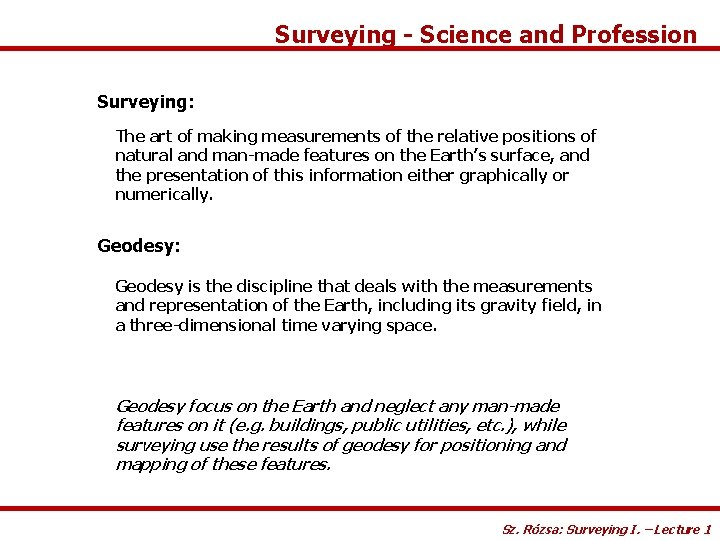Surveying - Science and Profession Surveying: The art of making measurements of the relative positions of natural and man-made features on the Earth’s surface, and the presentation of this information either graphically or numerically. Geodesy: Geodesy is the discipline that deals with the measurements and representation of the Earth, including its gravity field, in a three-dimensional time varying space. Geodesy focus on the Earth and neglect any man-made features on it (e. g. buildings, public utilities, etc. ), while surveying use the results of geodesy for positioning and mapping of these features. Sz. Rózsa: Surveying I. – Lecture 1Basic principles of Surveying Recall the definition of Surveying: The positioning is usually The art of making measurements of the relative positions of natural and man-made features on separated the Earth’sinto horizontal (2 D) and vertical surface, and the presentation of this information either (1 D) positioning. graphically or numerically. Nowadays 3 D positioning can be achieved using satellite techniques, too. How to achieve this? Let’s determine the position (XP, YP) of point P! Absolute vs Relative positioning XP d. BP P d. AP YP (XB, YB) Control points (known coords; marked on the field) (XA, YA) Sz. Rózsa: Surveying I. – Lecture 1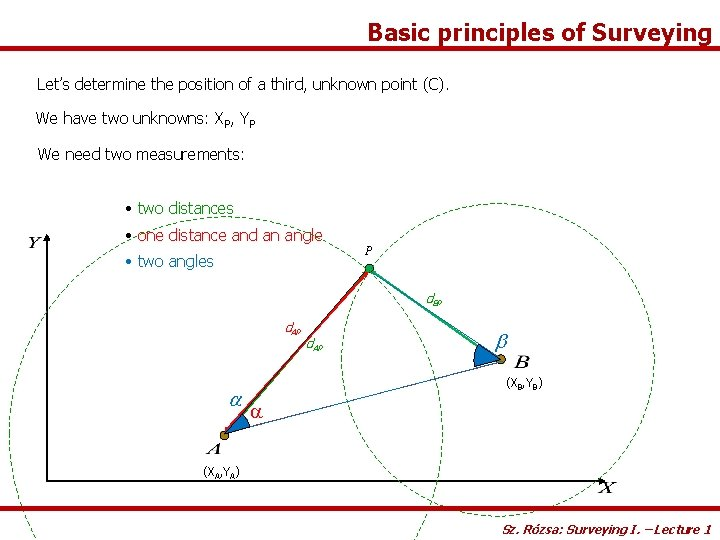Basic principles of Surveying Let’s determine the position of a third, unknown point (C). We have two unknowns: XP, YP b We need two measurements: a • two distances • one distance and an angle • two angles P d. BP d. AP a d. AP b (XB, YB) a (XA, YA) Sz. Rózsa: Surveying I. – Lecture 1Classification of Surveying Plane Surveying According to the space involved: • relatively small areas • surface of earth can supposed to be flat Note: The two radii can supposed to be parallel, when the l(A, B) is small. • measurements plotted represent a horizontal projection of the actual field measurements Sz. Rózsa: Surveying I. – Lecture 1Geodetic Surveying Classification of Surveying Don’t forget! Size does matter! • large areas • surface of the Earth can not supposed to be flat • the curvature of the Earth is taken into account Mostly used for establishing control networks, determining the size and shape of the Earth and determining the gravity field of the Earth. Sz. Rózsa: Surveying I. – Lecture 1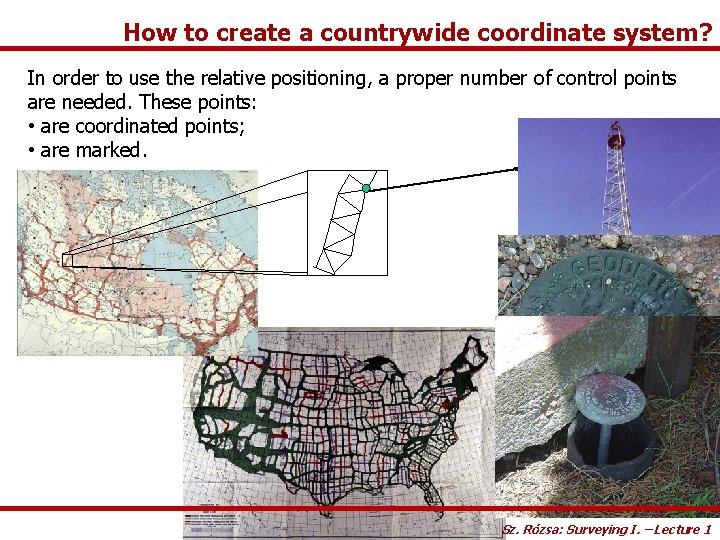How to create a countrywide coordinate system? In order to use the relative positioning, a proper number of control points are needed. These points: • are coordinated points; • are marked. Sz. Rózsa: Surveying I. – Lecture 1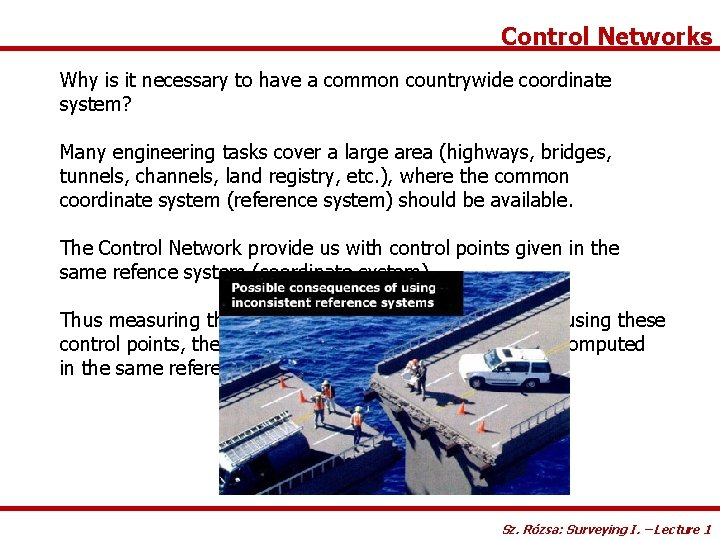Control Networks Why is it necessary to have a common countrywide coordinate system? Many engineering tasks cover a large area (highways, bridges, tunnels, channels, land registry, etc. ), where the common coordinate system (reference system) should be available. The Control Network provide us with control points given in the same refence system (coordinate system). Thus measuring the relative positions of unknown points using these control points, the coordinates of the new points can be computed in the same reference system. Sz. Rózsa: Surveying I. – Lecture 1The role of Surveying in Civil Engineering Practice Surveyors are needed: • to maintain the geometric order during the construction process • to provide fundamental data for the design and planning process • to provide quantity control during the construction process (for example: earthwork quantities) • to monitor the structure after the construction (subsidence, deformations, etc. ) What is this? Wrong geometry theappropriate structure is geometry, not functional! Laying them in–the outstanding structures can be created! Sz. Rózsa: Surveying I. – Lecture 1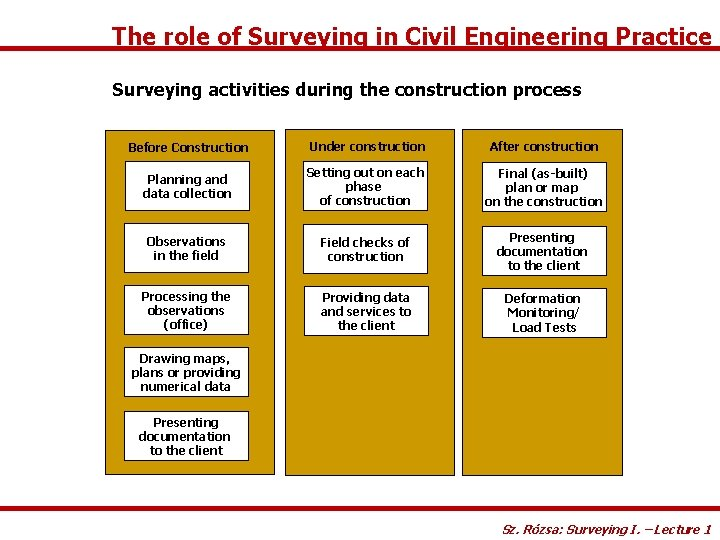The role of Surveying in Civil Engineering Practice Surveying activities during the construction process Before Construction Under construction After construction Planning and data collection Setting out on each phase of construction Final (as-built) plan or map on the construction Observations in the field Field checks of construction Presenting documentation to the client Processing the observations (office) Providing data and services to the client Deformation Monitoring/ Load Tests Drawing maps, plans or providing numerical data Presenting documentation to the client Sz. Rózsa: Surveying I. – Lecture 1Outline Introduction Historical Surveying - Science and Profession Methods of height determination Levelling The surveyors’ level Sz. Rózsa: Surveying I. – Lecture 1Methods of height determination Question 1: What does the height (elevation) of a point mean? Question 2: What does it mean, when point B is at a higher elevation than point A? Answer 1: The height of a point represents its energy level above a reference level. Answer 2: For example water flows from point B to point A. Sz. Rózsa: Surveying I. – Lecture 1Methods of height determination Definition of height systems: • The potential energy of a point should be represented by the height of a point. Hence water should flow from the higher elevation towards the lower elevation. • Should have metric unit. What should be the reference of height determination? What is the 0 level? • Since the height systems should represent the potential energy level, we need a reference surface, which is an equipotential surface of Earth’s gravity field. • The surface of calm water forms an equipotential surface • Mean Sea Level – Kronstadt (Baltic Sea) is used in Hungary (formerly Triest, Adriatic Sea). Sz. Rózsa: Surveying I. – Lecture 1Methods of height determination Equipotential surfaces equipotential surface g Gravity vector is always perpendicular to the equipotential surface. Equipotential surface (=) horizontal surface Gravity vector (=) vertical direction Sz. Rózsa: Surveying I. – Lecture 1Methods of height determination 1 D position determination - determining the height We can not determine absolute heights above the reference level Relative height determination - determining the height differences Levelling benchmarks are needed - control points for which the elevation is known. Reference level Sz. Rózsa: Surveying I. – Lecture 1Methods of height determination How can we determine the height difference? Two solutions: • setting a horizontal plane, and measuring the offset from this plane • measuring the slope and slope distance between the points Levelling Trigonometrical height determination Reference level Sz. Rózsa: Surveying I. – Lecture 1Outline Introduction Historical Surveying - Science and Profession Methods of height determination Levelling The surveyors’ level Sz. Rózsa: Surveying I. – Lecture 1The principle of levelling Line of sight d. A (l. A) d. B l. A equipo tential surfac e A l. B (l. B) DHAB B DHAB=l. A-l. B=(l. A)-d. A-(l. B)+d. B aphy topogr When d. A=d. B (spherical approximation, equal distance to A and B) DHAB=(l. A)-(l. B) Sz. Rózsa: Surveying I. – Lecture 2 1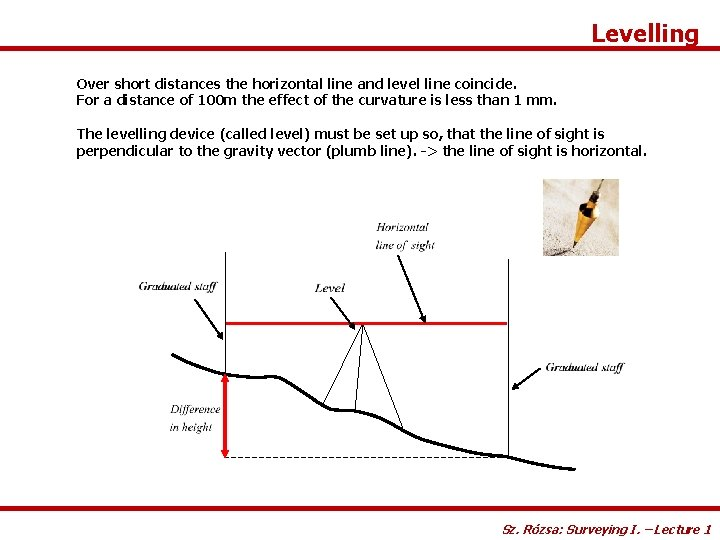Levelling Over short distances the horizontal line and level line coincide. For a distance of 100 m the effect of the curvature is less than 1 mm. The levelling device (called level) must be set up so, that the line of sight is perpendicular to the gravity vector (plumb line). -> the line of sight is horizontal. Sz. Rózsa: Surveying I. – Lecture 1Levelling Sz. Rózsa: Surveying I. – Lecture 1Outline Introduction Historical Surveying - Science and Profession Methods of height determination Levelling The surveyors’ level Sz. Rózsa: Surveying I. – Lecture 1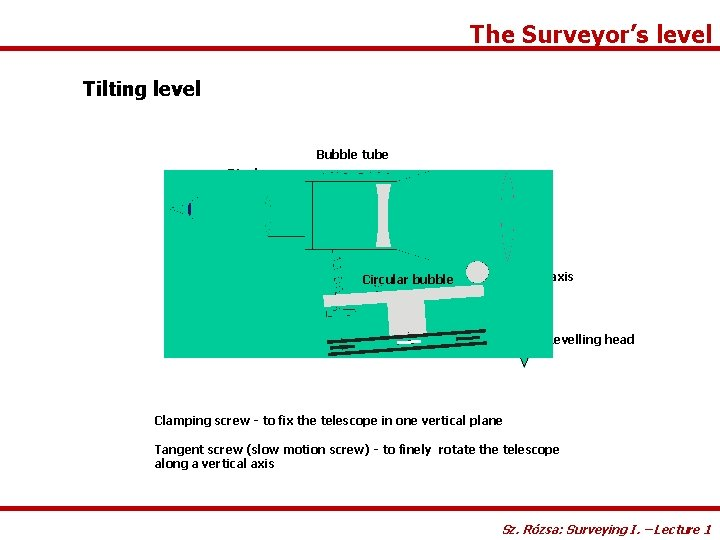The Surveyor’s level Tilting level Bubble tube Diaphragm Tilting screw Tilting axis Circular bubble Levelling head Clamping screw - to fix the telescope in one vertical plane Tangent screw (slow motion screw) - to finely rotate the telescope along a vertical axis Sz. Rózsa: Surveying I. – Lecture 1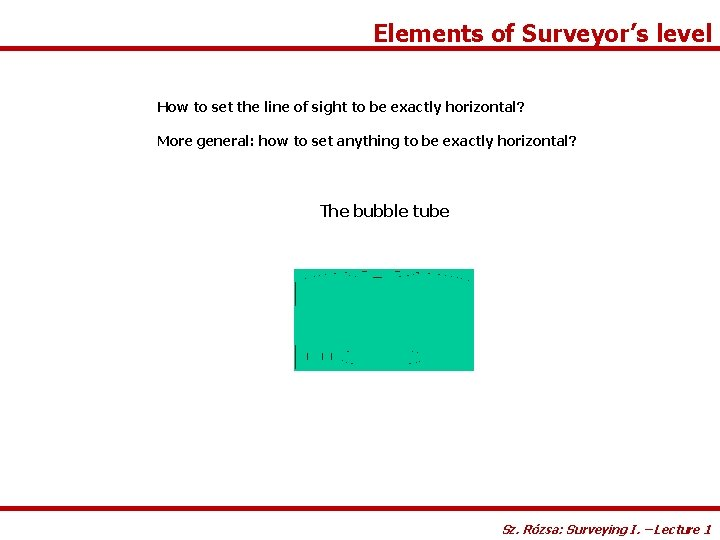Elements of Surveyor’s level How to set the line of sight to be exactly horizontal? More general: how to set anything to be exactly horizontal? The bubble tube Sz. Rózsa: Surveying I. – Lecture 1The bubble tube The radius determines the sensitivity of the bubble tube: Sensitivity: how much the bubble moves due to a given amount of inclination. The more the bubble moves, the more sensitive the bubble tube is. Sz. Rózsa: Surveying I. – Lecture 1The bubble tube The determination of sensitivity: Sz. Rózsa: Surveying I. – Lecture 1